# sql分组查询group by结合count，sum统计语句的实现（附带sql详细分析步骤）

15 篇文章 2 订阅

## 场景1：统计每个学生的加减分次数和总分

id自增编号bigint
student_name学生姓名varchar
score分数double
sort分数类型int1-加分；2-减分；
is_delete删除标志位int默认为0；

SELECT
student_name,
count(sort = 1 OR NULL) add_count,
count(sort = 2 OR NULL) sub_count,
sum(IF(sort = 1, score, 0)) add_score,
sum(IF(sort = 2, score, 0)) sub_score
FROM
student_score
WHERE
is_delete = 0
GROUP BY
student_name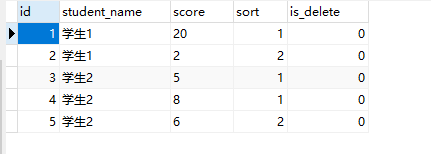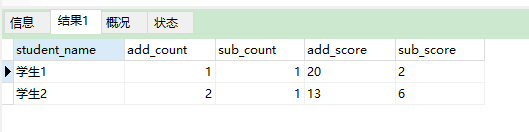SELECT
student_name,
count(case when sort = 1 then 1 else null end) add_count,
count(case when sort = 2 then 1 else null end) sub_count,
sum(case when sort = 1 then score else 0 end) add_score,
sum(case when sort = 2 then score else 0 end) sub_score
FROM
student_score
WHERE
is_delete = 0
GROUP BY
student_name


## 场景2：按照权重统计每个学生的体测成绩

800米30
50米15

id自增编号bigint
index_code指标编号varchar
index_name指标名称varchar
share指标权重int
is_delete删除标志位int默认为0；

id自增编号bigint
student_name学生名称varchar
index_code指标编号varchar
score对应指标得分double
is_delete删除标志位int默认为0；

SELECT
student_name,
index_code,
round(avg(score), 1) index_avg
FROM
score_record r
WHERE
r.is_delete = 0
GROUP BY
student_name, index_code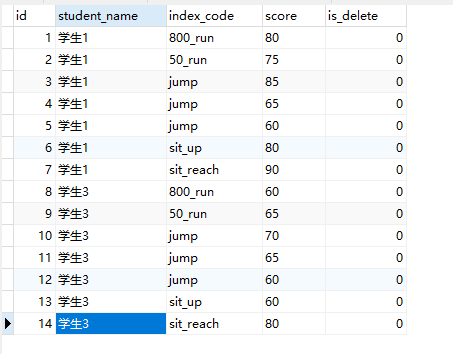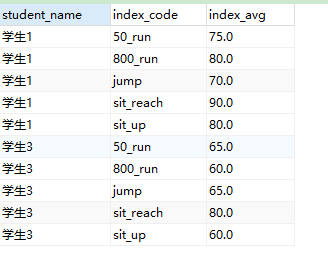SELECT
round(sum(s.share * t.index_avg) / 100, 2) score, t.student_name
FROM
(
SELECT
student_name,
index_code,
round(avg(score), 1) index_avg
FROM
score_record r
WHERE
r.is_delete = 0
GROUP BY
student_name,index_code
) t
LEFT JOIN index_score s ON t.index_code = s.index_code
GROUP BY
t.student_name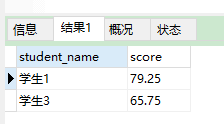08-219421
01-1786112-227434
05-3145
05-0614万+
04-262万+
07-262万+
06-203万+
12-311309
11-102万+
03-261万+
06-051824
01-035万+
02-252万+
05-236293
05-292万+
01-19440
04-045016
10-101万+点击重新获取扫码支付余额充值